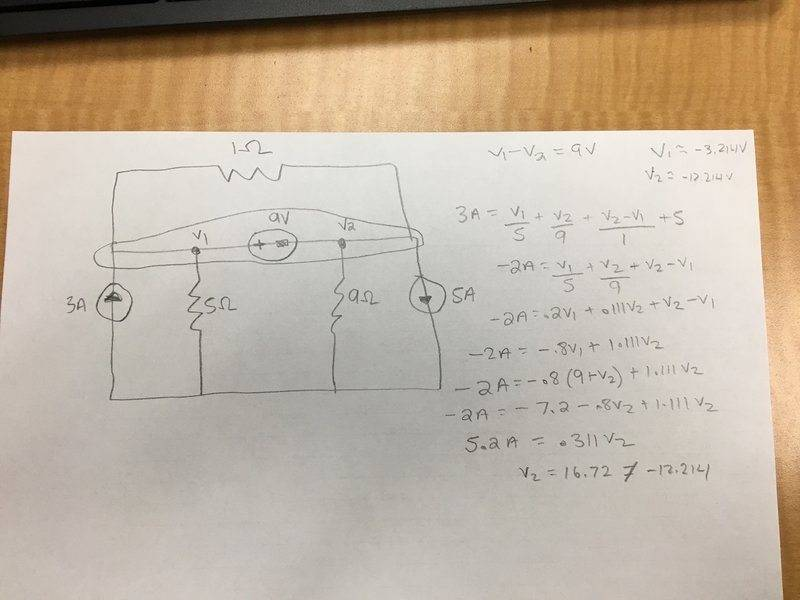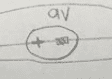# Solve Using Method of Node Voltages

## Homework Statement[/B]

## Homework Equations

This problem was from a practice exam. I know what V1 and V2 should be but can't get my answer to match. V1 should be -3.214 V and V2 should be -12.214 V

## The Attempt at a Solution

See attached picture for my work. [/B]

## Answers and RepliesWhat is this thing?

View attachment 212944
What is this thing?
That is a voltage source.

donpacino
Gold Member
I see your problem. It involves the 1 ohm resistor...
Hint: There is a voltage source in parallel with the resistor.

There are two ways you can look at your error. Either you should not have added the (V1-v2)/1 term, because the current though the 1 ohm resistor in no way effects V1 and V2. The other way you can look at it, is you need to write the equations for every current path in and out of the super node, in which case you missed (v2-v1)/1/

•CWatters
cnh1995
Homework Helper
Gold Member
I see your problem. It involves the 1 ohm resistor...
Hint: There is a voltage source in parallel with the resistor.

There are two ways you can look at your error. Either you should not have added the (V1-v2)/1 term, because the current though the 1 ohm resistor in no way effects V1 and V2. The other way you can look at it, is you need to write the equations for every current path in and out of the super node, in which case you missed (v2-v1)/1/
So I re did the problem taking the (v2-v1)/1 ohm out of the problem and got the right answer. My question is, why don't we need to account for this?

donpacino
Gold Member
So I re did the problem taking the (v2-v1)/1 ohm out of the problem and got the right answer. My question is, why don't we need to account for this?

There is a voltage source in parallel with the resistor. there will always be 9 amps going through the resistor, regardless of the other circuit conditions. It has no dependence on the value of V1 or V2

There are two current paths going to the resistor from your supernode. one going from V1 through the resistor counterclockwise, and one going from v2 through the resistor counterclockwise. You missed the counterclockwise one. If you were to write both equations, they would cancel each other out. Once you learn this will happen, you can just leave it out from the beginning.

There is a voltage source in parallel with the resistor. there will always be 9 amps going through the resistor, regardless of the other circuit conditions. It has no dependence on the value of V1 or V2

There are two current paths going to the resistor from your supernode. one going from V1 through the resistor counterclockwise, and one going from v2 through the resistor counterclockwise. You missed the counterclockwise one. If you were to write both equations, they would cancel each other out. Once you learn this will happen, you can just leave it out from the beginning.
Okay that makes sense thanks. One more quick question... Is the voltage across that 5 ohm resistor also -3.214 V or would it be positive 3.214 V ?

donpacino
Gold Member
Okay that makes sense thanks. One more quick question... Is the voltage across that 5 ohm resistor also -3.214 V or would it be positive 3.214 V ?

Voltage is a differential measurement, like height. If V1 is -3 V, then common usage says the voltage drop across the resistor is -3V. But one could describe the drop in the other direction (drop from the bottom end to the top) in which case the voltage would be positive.

An analogy to height... You're at the top of a mountain. Someone asks how high, you say 3000 feet down (-3000), because you are measuring from the top to the bottom. But then you ask someone else how big it is. they reply 3000 up. It is all about perspective.